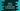# How to convert a decimal value to hexadecimal in Python## How to convert a decimal value to hexadecimal in Python:

In this post, we will learn different ways to convert a decimal value to hexadecimal using Python. Decimal number system is base 10 number system. We can use 0 to 9 to represent a decimal number. Hexadecimal number system is base 16 number system. We have to use 0 to 9 in hexadecimal to represent 0 to 9 decimal values and A to F in hexadecimal for 10 to 15 decimal values.

We can write our own function or we can use an inbuilt function hex to do the decimal to hexadecimal conversion.

Before we write our program, let’s understand the algorithm to do the conversion.

### Algorithm to convert a decimal to hexadecimal:

Let’s learn the algorithm to convert a decimal value to hexadecimal:

• Read the decimal value from the user.
• Divide the decimal number by 16.
• Store the remainder in hexadecimal.
• Keep the result and keep dividing the decimal by 16 until you get 0.
• If you combine the remainders in reverse, it will be the hexadecimal conversion.

### Example to convert decimal to hexadecimal:

Let me show you an example.

The following table shows the decimal and hexadecimal conversion of the digits:

0 0
1 1
2 2
3 3
4 4
5 5
6 6
7 7
8 8
9 9
10 A
11 B
12 C
13 D
14 E
15 F

Now, let’s convert 761 to hexadecimal:

• 761/16, the quotient is 47 and remainder is 9. 9 is 9 in hexadecimal
• 47/16, the quotient is 2 and remainder is 15. 15 is F in hexadecimal
• 2/16, the quotient is 0 and the remainder is 2. 2 is 2 in hexadecimal

If we combine the remainders in reverse, it is 2F9. So, the hexadecimal representation of 761 is 2F9.

### Python program 1: Convert decimal to hexadecimal by dividing the number repeatedly:

Let’s write it down in code. This program will take one number as input from the user and it will keep dividing the number by 16 until the quotient becomes 0.

It follows the same steps as we have discussed above.

``````hex_dict = {0: '0',
1: '1',
2: '2',
3: '3',
4: '4',
5: '5',
6: '6',
7: '7',
8: '8',
9: '9',
10: 'A',
11: 'B',
12: 'C',
13: 'D',
14: 'E',
15: 'F'}

num = int(input('Enter a number: '))

hex = ''

while(num > 0):
remainder = num % 16
hex = hex_dict[remainder] + hex
num = num // 16

Here,

• hex_dict is a dictionary with decimal keys and hexadecimal values. We will use this dictionary to get the hexadecimal value for the remainders.
• It asks the user to enter a number and stores it in the num variable.
• hex is an empty string variable to hold the hexadecimal string.
• The while loop is finding the hexadecimal value. It keeps running and finds the remainder by dividing the number by 16. It picks the hexadecimal value from the dictionary and appends it to the start of hex.
• Once the loop ends, it prints the hexadecimal value.

If you run this program, it will print output as like below:

``````Enter a number: 761

### Python program 2: Convert decimal to hexadecimal by using a separate method:

Let’s use a separate method to do the conversion.

``````def decimal_to_hex(num):
hex_dict = {0: '0',
1: '1',
2: '2',
3: '3',
4: '4',
5: '5',
6: '6',
7: '7',
8: '8',
9: '9',
10: 'A',
11: 'B',
12: 'C',
13: 'D',
14: 'E',
15: 'F'}

hex = ''

while(num > 0):
remainder = num % 16
hex = hex_dict[remainder] + hex
num = num // 16

return hex

num = int(input('Enter a number: '))

We created a method decimal_to_hex that takes a number as the parameter and returns the hexadecimal value. This is exactly the same program we discussed above. The only difference is that we are using a new method to do the calculation.

It will print a similar result.

``````Enter a number: 1023

### Python program 3: Convert decimal to hexadecimal by using a recursive method:

We can use a recursive method to convert a decimal to hexadecimal. Let’s take a look at the program:

``````hex_dict = {0: '0',
1: '1',
2: '2',
3: '3',
4: '4',
5: '5',
6: '6',
7: '7',
8: '8',
9: '9',
10: 'A',
11: 'B',
12: 'C',
13: 'D',
14: 'E',
15: 'F'}

def decimal_to_hex(num, hex):
if num == 0:
return hex

remainder = num % 16

return decimal_to_hex(num // 16, hex_dict[remainder] + hex)

num = int(input('Enter a number: '))

Here,

• decimal_to_hex is a recursive method. It takes a number and one string as the parameter.
• If the value of the number is 0, it will return the string.
• Else, it finds the remainder and calls decimal_to_hex again to find the hexadecimal value. It passes num // 16 as the number and appends the hexadecimal value to the front of the hex string as the second parameter.

If you run this program, it will print a similar result.

``````Enter a number: 1023

### Python program 4: Decimal to hexadecimal by using hex:

hex() is a built-in method that can be used to convert an integer to hexadecimal. This method takes an integer as the parameter and returns the hexadecimal value.

``hex(num)``

Where num is the number parameter. It returns the hexadecimal string. It throws TypeError if anything other than integer values are passed to hex.

``````num = int(input('Enter a number: '))